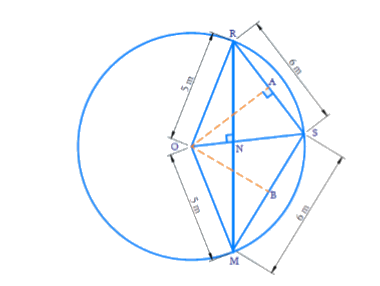# Ex.10.4 Q5 Circles Solution - NCERT Maths Class 9

## Question

Three girls Reshma, Salma and Mandip are playing a game by standing on a circle of radius $$5\rm\,m$$ drawn in a park. Reshma throws a ball to Salma, Salma to Mandip, Mandip to Reshma. If the distance between Reshma and Salma and between Salma and Mandip is $$6\,\rm m$$ each, what is the distance between Reshma and Mandip?

Video Solution
Circles
Ex 10.4 | Question 5

## Text Solution

What is known?

Three players are standing in a circle. Distance between two pairs is given. Radius of circle is given.

What is unknown?

Distance between Reshma and Mandip

Reasoning:

Perpendicular from center to either of the chord bisects the chord.Using this fact and by pythagoras theorem we can find value of $$OA$$. After that we can find area of $$∆ORS$$ by two ways like $$RS$$ as base and $$OA$$ as height or $$OS$$ as base and $$RN$$ as height. From this we will get the value of $$RN$$ and double of value $$RN$$ will will give the distance between Reshma and Mandip.

Steps:Let $$O$$ be the centre of the circle and $$R, M$$ and $$S$$ denote Reshma, Mandip and Salma respectively.

Draw a perpendicular $${OA}$$ to $${RS}$$ from $${O.}$$ Then \begin{align}{RA = AS = 3}\,\rm {m.} \end{align}

Using Pythagoras theorem we get, \begin{align}OA = 4 \text{m.} \end{align}

We can see that quadrilateral $$ORSM$$ takes that shape of a kite.

(Because $$OR = OM$$ and $$RS = SM$$).

We know that the diagonals of a kite are perpendicular and the main diagonal bisects the other diagonal.

$$\angle {RNS}$$ will be $$90^\circ$$ and \begin{align}{RN = NM} \end{align}

$$\text{Area of } ∆ORS$$ \begin{align} &= \frac{1}{2} \times RS \times OA\\ &=\frac{1}{2} \times 6 \times4\\&= 12 \dots(1)\end{align}

Also

$$\text{Area of} ∆ORS$$ \begin{align}& =\frac{1}{2} \times OS \times RN\\&= \frac{1}{2} \times 5 \times RN\dots(2)\end{align}

From equation ($$1$$) and ($$2$$)

\begin{align}\frac{1}{2} \times 5 \times RN\,= 12\end{align}

\begin{align}RN = \frac{{24}}{5} = 4.8\, \rm m\end{align}

\begin{align}RM = 2 × RN = 2 × 4.8 = 9.6 \, \rm m\end{align}

Distance between Reshma and Salma is $$9.6\, \rm{ m.}$$

Video Solution
Circles
Ex 10.4 | Question 5
Learn from the best math teachers and top your exams

• Live one on one classroom and doubt clearing
• Practice worksheets in and after class for conceptual clarity
• Personalized curriculum to keep up with school